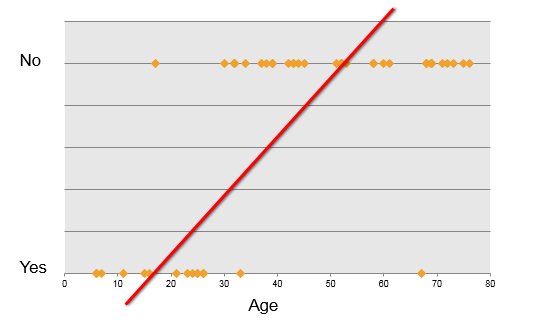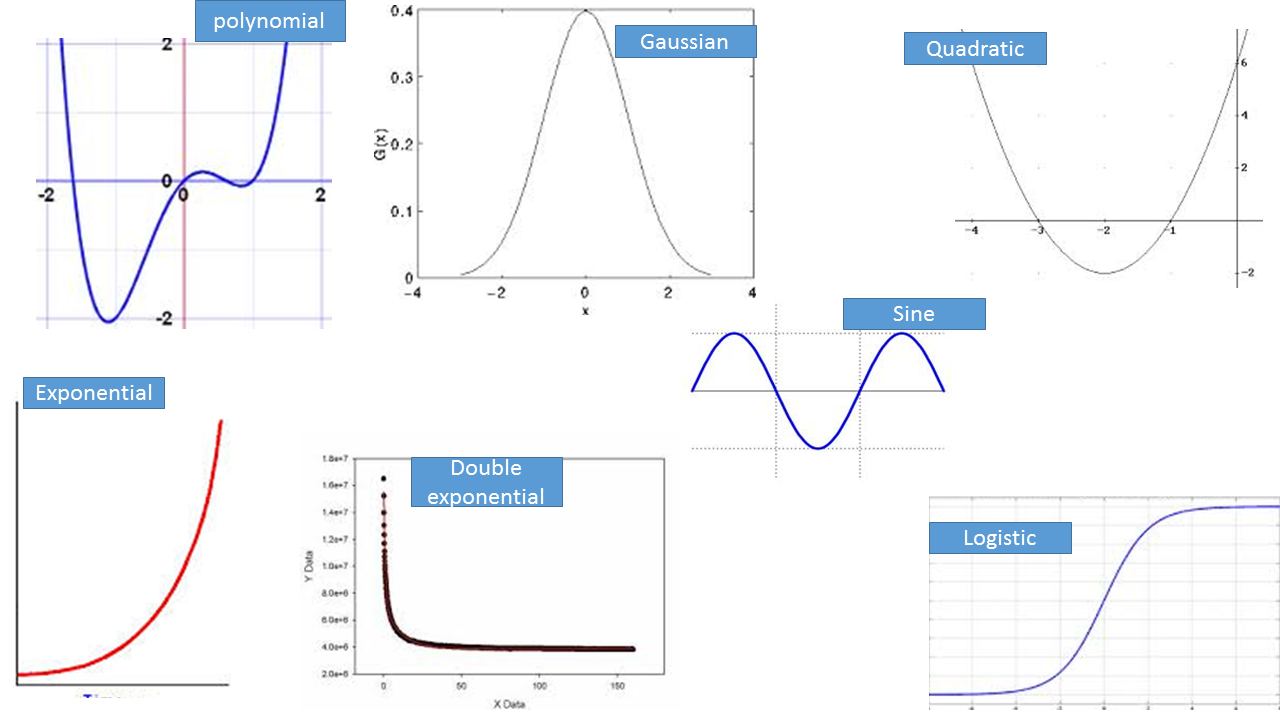• No products in the cart.

# 204.2.1 Logistic Regression, why do we need it?

##### What happens if Linear Regression doesn't work?

In this series we will try to explore Logistic Regression Models. For the starters we will do a recap of Linear Regression and see if it works all the time.

### Practice : What is the need of logistic regression?

• Dataset: Product Sales Data/Product_sales.csv
• What are the variables in the dataset?
• Build a predictive model for Bought vs Age
• What is R-Square?
• If Age is 4 then will that customer buy the product?
• If Age is 105 then will that customer buy the product?
In :
```import pandas as pd
```
In :
```#What are the variables in the dataset?
sales.columns.values
```
Out:
`array(['Age', 'Bought'], dtype=object)`
In :
```#Build a predictive model for Bought vs Age

### we need to use the statsmodels package, which enables many statistical methods to be used in Python
import statsmodels.formula.api as sm
from statsmodels.formula.api import ols
model = sm.ols(formula='Bought ~ Age', data=sales)
fitted = model.fit()
fitted.summary()
```
Out:
Dep. Variable: R-squared: Bought 0.842 OLS 0.842 Least Squares 2480. Sun, 16 Oct 2016 1.63e-188 14:35:39 95.589 467 -187.2 465 -178.9 1 nonrobust
coef std err t P>|t| [95.0% Conf. Int.] -0.1704 0.015 -11.156 0.000 -0.200 -0.140 0.0209 0.000 49.803 0.000 0.020 0.022
 Omnibus: Durbin-Watson: 77.279 1.362 0 1022.09 0.056 1.14e-222 10.247 60.7
In :
```#What is R-Square?
fitted.rsquared
```
Out:
`0.84212439295277375`
In :
```#If Age is 4 then will that customer buy the product?

from sklearn.linear_model import LinearRegression
lr = LinearRegression()
lr.fit(sales[["Age"]], sales[["Bought"]])

age1=4
predict1=lr.predict(age1)
predict1
```
Out:
`array([[-0.08664394]])`
In :
```age2=105
predict2=lr.predict(age2)
predict2
```
Out:
`array([[ 2.02851132]])`

### Something went wrong

• The model that we built above is not right.
• There is certain issues with the type of dependent variable.
• The dependent variable is not continuous it is binary.
• We can’t fit a linear regression line to this data.

### Why not linear ?

• Consider Product sales data. The dataset has two columns.
• Age – continuous variable between 6-80### Real-life examples

• Gaming – Win vs. Loss
• Marketing – Response vs. No Response
• Credit card & Loans – Default vs. Non Default
• Operations – Attrition vs. Retention
• Websites – Click vs. No click
• Fraud identification – Fraud vs. Non Fraud
• Healthcare – Cure vs. No Cure

The output of these non linear functions cannot be justifies with a linear model.

### Some Nonlinear Functions21st June 2017